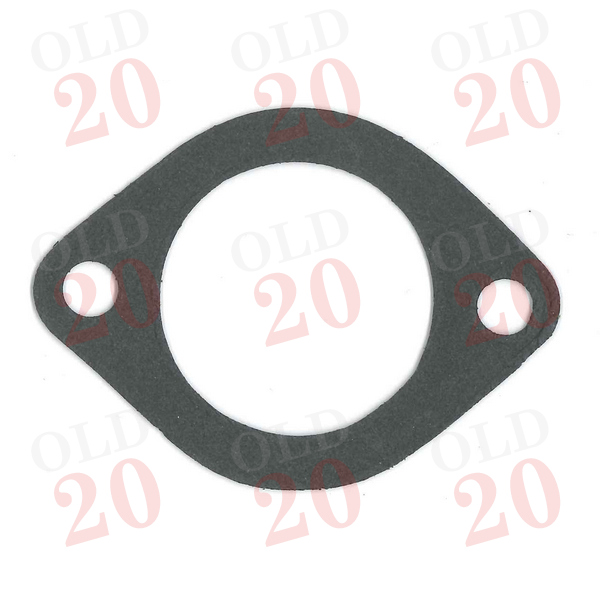View larger

1210050

New

£ 1.20Tractors < Ferguson < TEA20
Tractors < Ferguson < TED20
Tractors < Ferguson < TEF20
Tractors < Ferguson < FE35 (87mm)
Tractors < Ferguson < FE35 (23c)
Tractors < Landini < 30 < 5830
Tractors < Landini < 30 < 6030
Tractors < Landini < 40 < 5840
Tractors < Landini < 500 < 5500
Tractors < Landini < 60 < 5560
Tractors < Landini < 60 < 5860
Tractors < Landini < 60 < 6060
Tractors < Landini < 70 < 5870
Tractors < Landini < 70 < 6070
Tractors < Landini < Blizzard < Blizzard 50
Tractors < Massey Ferguson < Pre-100 Series < 25
Tractors < Massey Ferguson < Pre-100 Series < 35
Tractors < Massey Ferguson < Pre-100 Series < 35X
Tractors < Massey Ferguson < 100 < 122
Tractors < Massey Ferguson < 100 < 130
Tractors < Massey Ferguson < 100 < 133
Tractors < Massey Ferguson < 100 < 134C
Tractors < Massey Ferguson < 100 < 135
Tractors < Massey Ferguson < 100 < 140
Tractors < Massey Ferguson < 100 < 145
Tractors < Massey Ferguson < 100 < 148
Tractors < Massey Ferguson < 100 < 150
Tractors < Massey Ferguson < 100 < 152
Tractors < Massey Ferguson < 100 < 154
Tractors < Massey Ferguson < 200 < 230
Tractors < Massey Ferguson < 200 < 231
Tractors < Massey Ferguson < 200 < 233
Tractors < Massey Ferguson < 200 < 234C
Tractors < Massey Ferguson < 200 < 235
Tractors < Massey Ferguson < 200 < 240
Tractors < Massey Ferguson < 200 < 243
Tractors < Massey Ferguson < 200 < 244CF
Tractors < Massey Ferguson < 200 < 245
Tractors < Massey Ferguson < 200 < 250
Tractors < Massey Ferguson < 200 < 253
Tractors < Massey Ferguson < 200 < 254
Tractors < Massey Ferguson < 200 < 255
Tractors < Massey Ferguson < 200 < 263
Tractors < Massey Ferguson < 300 < 333
Tractors < Massey Ferguson < 300 < 340
Tractors < Massey Ferguson < 300 < 342
Tractors < Massey Ferguson < 300 < 350
Tractors < Massey Ferguson < 300 < 352
Tractors < Massey Ferguson < 300 < 353
Tractors < Massey Ferguson < 300 < 353LX
Tractors < Massey Ferguson < 300 < 355
Tractors < Massey Ferguson < 300 < 356CF
Tractors < Massey Ferguson < 300 < 360
Tractors < Massey Ferguson < 300 < 363
Tractors < Massey Ferguson < 500 < 550
Tractors < Massey Harris < Vintage < 50
Tractors < Perkins < Vintage < P6

Supplier Reference :0490033, 10490184, 3910266M1, 1650482M1, 825659M1, 4222057M1, 36834169

 Section Cooling
This simple gasket is used for the top and the bottom of the thermostat housing on Ferguson T20 & MF tractors.

You can also use this gasket for the top of the Perkins P6 waterpump.